Important Questions: Comparing Quantities

# Important Questions: Comparing Quantities

Test Description

## 20 Questions MCQ Test Mathematics (Maths) Class 7 | Important Questions: Comparing Quantities

Important Questions: Comparing Quantities for Class 7 2022 is part of Mathematics (Maths) Class 7 preparation. The Important Questions: Comparing Quantities questions and answers have been prepared according to the Class 7 exam syllabus.The Important Questions: Comparing Quantities MCQs are made for Class 7 2022 Exam. Find important definitions, questions, notes, meanings, examples, exercises, MCQs and online tests for Important Questions: Comparing Quantities below.
Solutions of Important Questions: Comparing Quantities questions in English are available as part of our Mathematics (Maths) Class 7 for Class 7 & Important Questions: Comparing Quantities solutions in Hindi for Mathematics (Maths) Class 7 course. Download more important topics, notes, lectures and mock test series for Class 7 Exam by signing up for free. Attempt Important Questions: Comparing Quantities | 20 questions in 20 minutes | Mock test for Class 7 preparation | Free important questions MCQ to study Mathematics (Maths) Class 7 for Class 7 Exam | Download free PDF with solutions
 1 Crore+ students have signed up on EduRev. Have you?
Important Questions: Comparing Quantities - Question 1

###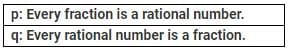Which of the following is correct?

Detailed Solution for Important Questions: Comparing Quantities - Question 1

Let us consider a fraction 3/5 and a rational number = −4/7 By definition, 3/5 is a rational number.
So, p is true. By definition, −4/7 is not a fraction, since, −4  is not a natural number. So, q is false.

Important Questions: Comparing Quantities - Question 2

### Which of the following is not a rational number(s)?

Detailed Solution for Important Questions: Comparing Quantities - Question 2

A rational number is defined as the number which can be expressed in the form of p/q, where p and q are integers and q ≠ 0.

√2  is not an integer.

Hence, √2/3 is not a rational number.

Important Questions: Comparing Quantities - Question 3

### p: All integers are rational numbers. q: Every rational number is an integer. Which of the following statements is correct?

Detailed Solution for Important Questions: Comparing Quantities - Question 3

Since, every integer having a denominator 1 can be expressed in P/q  form, p is true.
Since, a rational number with denominator other than 1 is not an integer (e.g., 3/5 ), q is false.

Important Questions: Comparing Quantities - Question 4

What type of a number is −3/0?

Detailed Solution for Important Questions: Comparing Quantities - Question 4

Since, denominator is 0, it is not a rational number.

Important Questions: Comparing Quantities - Question 5

Which among the following is a rational number equivalent to 5/3?

Important Questions: Comparing Quantities - Question 6

What type of a numerator does 0/7 have?

Important Questions: Comparing Quantities - Question 7

Which of the following statements is true?

Important Questions: Comparing Quantities - Question 8

If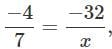what is the value of x?

Important Questions: Comparing Quantities - Question 9

Which is the greatest?

Detailed Solution for Important Questions: Comparing Quantities - Question 9

Since we know , greater the denominator smaller is the number, but since all numbers are negative, the number nearer to zero will be greatest ,so -5/17 is the greatest number.

Important Questions: Comparing Quantities - Question 10

Which is the correct descending order of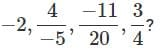Detailed Solution for Important Questions: Comparing Quantities - Question 10

L.C.M. of 5, 4 and 20 is 20.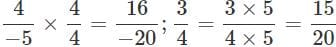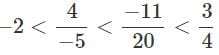or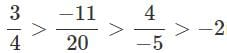is the required descending order. Rewrite equivalent fractions of the given fractions and then compare then. Arrange them in descending order.

Important Questions: Comparing Quantities - Question 11

What is the average of the two middle rational numbers if 4/7,1/3,2/5 and 5/9 are arranged in ascending order?

Detailed Solution for Important Questions: Comparing Quantities - Question 11

The ascending order of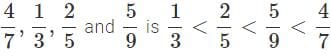The two middle numbers are 2/5 and 5/9
∴  Average
=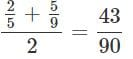Important Questions: Comparing Quantities - Question 12

What is the percentage of the least number in the greatest number of 3/5,9/5,1/5  and 7/5 ?

Detailed Solution for Important Questions: Comparing Quantities - Question 12

The given numbers can be arranged in ascending order as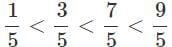The greatest number = 9/5; The least number = 1/5
We have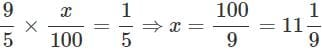Important Questions: Comparing Quantities - Question 13

What is the difference between the greatest and least numbers of 5/9,1/9 and 11/9 ?

Detailed Solution for Important Questions: Comparing Quantities - Question 13

The ascending order of given numbers is 1/9,5/9,11/9.
∴  Required difference 11/9−1/9 = 10/9

Important Questions: Comparing Quantities - Question 14

Which of the following pairs represent the same rational number?

Detailed Solution for Important Questions: Comparing Quantities - Question 14

−24/15  is the equivalent rational number of −8/5.

Important Questions: Comparing Quantities - Question 15

P: The quotient of two integers is always a rational number.
Q : 1/0 is not rational. Which of the following statements is true?

Detailed Solution for Important Questions: Comparing Quantities - Question 15

Since, 1/0 is not rational, the quotient of two integers is not always rational.

Important Questions: Comparing Quantities - Question 16

What is the result of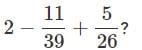Detailed Solution for Important Questions: Comparing Quantities - Question 16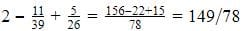Important Questions: Comparing Quantities - Question 17

Which is the equivalent of -143/21

Important Questions: Comparing Quantities - Question 18

Of which property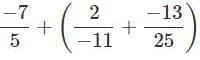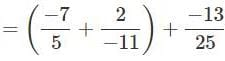is an example?

Important Questions: Comparing Quantities - Question 19

Which of the following statements is correct?

Detailed Solution for Important Questions: Comparing Quantities - Question 19

All the given statements are correct. Consider 4/5 [a]
Since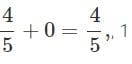is the additive identity of rational numbers. [b]
Since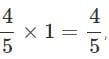is the  multiplicative identity of rational numbers. [c]
Since 0+0 = 0,0 is the additive inverse of 0.

Important Questions: Comparing Quantities - Question 20

The sum of two rational numbers is −3. If one of the numbers is −7/5, find the other number.

Detailed Solution for Important Questions: Comparing Quantities - Question 20

Let x be the required number. So,

x+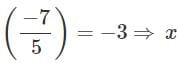=−3+ 7/5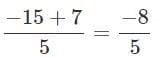## Mathematics (Maths) Class 7

168 videos|252 docs|45 tests
 Use Code STAYHOME200 and get INR 200 additional OFF Use Coupon Code
Information about Important Questions: Comparing Quantities Page
In this test you can find the Exam questions for Important Questions: Comparing Quantities solved & explained in the simplest way possible. Besides giving Questions and answers for Important Questions: Comparing Quantities, EduRev gives you an ample number of Online tests for practice

## Mathematics (Maths) Class 7

168 videos|252 docs|45 tests